TUTORIAL QUESTIONS 130-140

Questions:
130: Motion of a standing wave tied at both ends
135: Motion of a standing wave tied at both ends
137: Fundamental frequency of a standing wave
140: Motion of a standing wave loose at one end

130.  Consider the diagram below for a standing wave on a string 12 ft long that is tied at both ends.  The wave velocity in the string is 16 ft/s.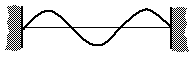What will the string look like exactly 1.25 seconds after the snapshot above?
a)b)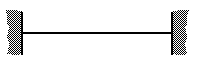c)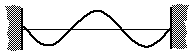d)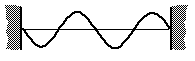e) None of the above

Fundamentals of Sound reference: Sec. 2-C.

135.  Consider the diagram below for a standing wave on a string 12 ft long that is tied at both ends.  The wave velocity in the string is 16 ft/s.Exactly how many seconds immediately after the above snapshot will the string look like this:a) 1/8 s        b) 1/4 s        c) 3/8 s
d) 1/2 s        e) 3/4 s        f) 1 s
g) 2 s           h) 12 s          i) 16 s

Fundamentals of Sound reference: Sec. 2-C.

137. How could I increase the fundamental frequency of a violin string attached at both ends?
a) Tighten the string
b) Shorten the string
c) Move the violin bow on the string faster
d) Both a) and b)
e)  a), b), and c)

Fundamentals of Sound reference: Sec. 2-C.

.

140.  Consider the following standing wave in a medium that is closed at the left end and open at the right end.  The wave velocity in this medium is 4 ft/s, and the medium is 14 ft long.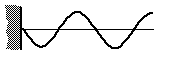Which mode is this?
a) 3rd harmonic
b) 4th harmonic
c) 5th harmonic
d) 2nd overtone
e) none of the above

Fundamentals of Sound reference: Sec. 2-D.

Back to Tutorial Listing